# MR Second Printing

## Contents

### Chapter 1

• p3, middle: convenient way to calculate a lower bound on the dof --> convenient way to calculate the dof

### Chapter 2

• Proposition 2.2, p16: This formula holds only if all joint constraints are independent. If they are not independent then the formula provides a lower bound on the number of degrees of freedom --> This formula holds in "generic" cases, but it fails under certain configurations of the links and joints, such as when the joint constraints are not independent.
• Chapter 2.2.2, Example 2.3, p16: "Substituting" is misspelled.
• Eliminate two sentences on p19: "Grubler's formula provides ... are discussed in Chapter 7."
• Fifth bullet of Chapter 2.6 p32: Eliminate the last sentence "If the constraints enforced ... degrees of freedom."

### Chapter 3

• In the caption of Figure 3.3 p53,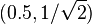$(0.5,1/\sqrt{2})$ should read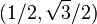$(1/2, \sqrt{3}/2)$, and$(-1/\sqrt{2},0.5)$ should read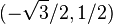$(-\sqrt{3}/2, 1/2)$.
• Under "Representing a configuration" in Chapter 3.3.1.2, the text says "Tbc = (Rbc,pbc) represents {b} relative to {c}" but it should say "represents {c} relative to {b}".
• There is a typo in the final matrix, Tce, of Example 3.19. The term$130/\sqrt{2}$ should be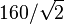$160/\sqrt{2}$. (Also, there is no need to use Tad in this example.)
• In the displayed equation just after Equation (3.76), the left-hand side should be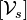$[\mathcal{V}_s]$ (the brackets are missing).
• Exercise 3.16(i) asks for "the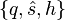$\{q,\hat{s},h\}$ representation" but it should say "a$\{q,\hat{s},h\}$ representation" since q is not unique.
• Near the end of Chapter 3.6 Software, the function "AxisAng" should be written "AxisAng6."

### Chapter 4

• Example 4.1, last row of the table (screw axis for joint 3): The linear component vi should be (0, − L2,0) (the sign is missing).

### Chapter 5

• Equation (5.7): The the first two terms on the right-hand side of the equation should be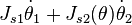$J_{s1} \dot{\theta}_1 + J_{s2}(\theta) \dot{\theta}_2$.
• Exercise 5.2(b): The wrench applied by the last link, expressed in the {s} frame, is 5 N in the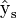$\hat{\text{y}}_{\text{s}}$ direction, with zeros in all other components. (The wording seems to imply that the force is through the tip of the last link, which is incorrect.)
• Exercise 5.16 and Figure 5.26: The robot is referred to as PRRRRR, but it is PRPRRR.
• Figure 5.15(b): The circular arrow indicating the rotation of joint 3 is slightly misplaced.
• Exercise 5.25: This problem instructs you to use the space Jacobian, so you should use it for the manipulability ellipsoids in this problem (even though it is generally preferred to use the body Jacobian for manipulability ellipsoids).

### Chapter 6

• Chapter 6.3, first line after Eq (6.7): the matrices$T_{sd}^{-1} \dot{T}_{sd}$ and$\dot{T}_{sd} T_{sd}^{-1}$ are referred to as twists, but these are the se(3) matrix representations of the twists.

### Chapter 7

• Just below caption for Figure 7.8: There is an extraneous dot.

### Chapter 8

• In Equation (8.14),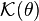$\mathcal{K}(\theta)$ should be replaced by$\mathcal{K}(\theta,\dot{\theta})$.
• Figure 8.5 says the volume of the rectangular parallelepiped is abc but it should be hlw.
• End of first sentence after Eq (8.26) in Section 8.2.1 should read "columns of Rbc correspond to the eigenvectors of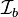$\mathcal{I}_b$." (The word "eigenvalues" should be replaced by "eigenvectors.")
• Caption of Figure 8.10: The operating region is light gray and the continuous operating region is dark gray.
• Exercise 8.6(a): The expression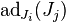$\text{ad}_{J_i}(J_j)$ has the indices switched; the correct expression is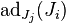$\text{ad}_{J_j}(J_i)$.
• Exercise 8.7: The expression should be written: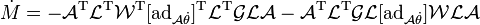$\dot{M} = -\mathcal{A}^{\rm T} \mathcal{L}^{\rm T} \mathcal{W}^{\rm T} [\mbox{ad}_{\mathcal{A} \dot{\theta}}]^{\rm T} \mathcal{L}^{\rm T} \mathcal{GLA} - \mathcal{A}^{\rm T} \mathcal{L}^{\rm T} \mathcal{GL} [\mbox{ad}_{\mathcal{A} \dot{\theta}}] \mathcal{WLA}$

### Chapter 10

• Equation (10.4) should read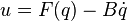$u = F(q) - B \dot{q}$ (the plus sign in the book should be a minus sign).

### Chapter 11

• In the displayed equation after Equation (11.18), the vector Xe(t) is a six-vector. The bottom three elements are written correctly, but the top three elements, an angular velocity, are written instead in their 3x3 so(3) form. Also, the term written RT(d) should be written RT(t).
• In Equation (11.33), the right-hand side should be zero (c = 0).
• Figure 11.24, the Robonaut 2 series elastic actuator, 4th sentence of the caption: The words "outer" and "inner" should be switched, so the new sentence reads "The inner ring of hole mounts connects to the harmonic gearhead output, and the outer ring of hole mounts is the output of the SEA, connecting to the next link."

### Chapter 12

• In Example 12.4, the elements of the wrenches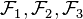$\mathcal{F}_1, \mathcal{F}_2, \mathcal{F}_3$ are erroneously written in the order (fx,fy,mz); they should be written in the order (mz,fx,fy), i.e., ( − 2,0,1),(1, − 1,0),(1,1,0), respectively.
• Caption of Figure 12.14, second-to-last sentence: "rotation if possible" should be "rotation is possible."

### Chapter 13

• Equation (13.29): yp should be yP.

### Appendix C

• Section C.3, Equation (C.5): All instances of ϕi − 1 should be replaced by ϕi.
• In Equation (C.12), two instances of Mi − 1 should be replaced by Mi.

### A partial list of errata contributors

Thanks to the following people who provided corrections, starting from the preliminary version of the book posted in October, 2016:

H. Andy Nam, Eric Lee, Yuchen Rao, Chainatee Tanakulrongson, Mengjiao Hong, Kevin Cheng, Jens Lundell, Elton Cheng, Michael Young, Jarvis Schultz, Logan Springgate, Sofya Akhmametyeva, Aykut Onol, Josh Holcomb, Yue Chen, Mark Shi, AJ Ibraheem, Yalun Wen, Seongjae Jeong, Josh Mehling, Felix Wang, Drew Warren, Chris Miller, Clemens Eppner, Zack Woodruff, Jian Shi, Jixiang Zhang, Shachar Liberman, Will Wu, Dirk Boysen, Awe Wang, Ville Kyrki, John Troll, Andrew Taylor, Nikhil Bakshi